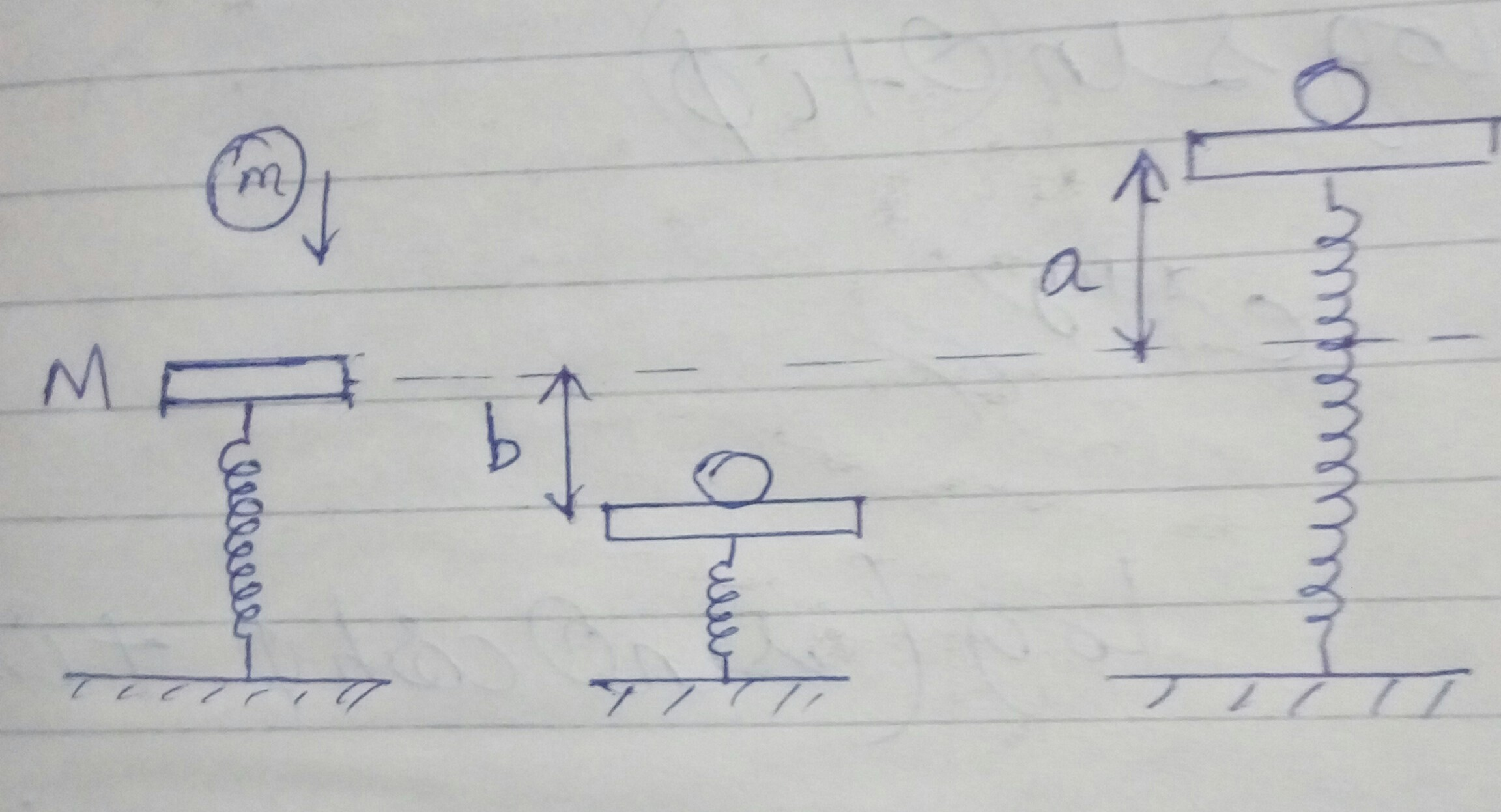# A question

A mass $M$ is in static equilibrium on a massless vertical spring as shown. A ball of mass m dropped from certain height sticks to the mass $M$ after colliding with it. The oscillations they perform reach to height 'a' above original level of scales and depth $b$ below it.

a) Find time period of oscillations.
b) What is the height above the initial level from which the mass m was dropped?Note by Tanishq Varshney
4 years, 10 months ago

This discussion board is a place to discuss our Daily Challenges and the math and science related to those challenges. Explanations are more than just a solution — they should explain the steps and thinking strategies that you used to obtain the solution. Comments should further the discussion of math and science.

When posting on Brilliant:

• Use the emojis to react to an explanation, whether you're congratulating a job well done , or just really confused .
• Ask specific questions about the challenge or the steps in somebody's explanation. Well-posed questions can add a lot to the discussion, but posting "I don't understand!" doesn't help anyone.
• Try to contribute something new to the discussion, whether it is an extension, generalization or other idea related to the challenge.

MarkdownAppears as
*italics* or _italics_ italics
**bold** or __bold__ bold
- bulleted- list
• bulleted
• list
1. numbered2. list
1. numbered
2. list
Note: you must add a full line of space before and after lists for them to show up correctly
paragraph 1paragraph 2

paragraph 1

paragraph 2

[example link](https://brilliant.org)example link
> This is a quote
This is a quote
    # I indented these lines
# 4 spaces, and now they show
# up as a code block.

print "hello world"
# I indented these lines
# 4 spaces, and now they show
# up as a code block.

print "hello world"
MathAppears as
Remember to wrap math in $$ ... $$ or $ ... $ to ensure proper formatting.
2 \times 3 $2 \times 3$
2^{34} $2^{34}$
a_{i-1} $a_{i-1}$
\frac{2}{3} $\frac{2}{3}$
\sqrt{2} $\sqrt{2}$
\sum_{i=1}^3 $\sum_{i=1}^3$
\sin \theta $\sin \theta$
\boxed{123} $\boxed{123}$

Sort by:

At the time when the body sticks to the pan of the spring, $\ddot y = \dfrac m{m+M}g - \dfrac\chi {m+M}y$ Here, downwards is taken to be positive.

At the equilibrium position, $\ddot y=0$

So, $y_{eq} = \dfrac{mg}\chi,\text{ downwards}$

Also, $\dfrac{a+b}2 = A,\text{ the amplitude}$

So $y_{eq} + b = A = \dfrac{a+b }2\\\Rightarrow \dfrac{mg}\chi = \dfrac{b-a}2\\\chi = \dfrac{2mg}{b-a}$

As we know, $T = 2\pi \sqrt{\dfrac{m+M}\chi}=2\pi\sqrt{\dfrac{(b-a)(m+M)}{2mg}}$

Now, to find $h$,$Mg = ky_0$\begin{aligned}mg(h+b) + Mgb &= \dfrac k2(y_0+b)^2-\dfrac k2 y_0^2\\ &=\dfrac k 2 (b^2+2by_0)\end{aligned}\\ \boxed{mg(h+b) = \dfrac k 2 b^2} \begin{aligned}mg(h-a)-Mga &= \dfrac k 2 (a-y_0)^2-\dfrac k 2 y_0^2\\&=\dfrac k 2 (a^2-2ay_0)\end{aligned} \\ \boxed{mg(h-a)=\dfrac k 2 a^2} Dividing, $\dfrac{h+b}{h-a} = \dfrac{b^2}{a^2} \\h+b = \dfrac{b^2}{b-a}\\h = \dfrac{ab}{b-a}$

- 4 years, 10 months ago

yup it will , thank you once again , but I feel the answer for h is not correct.

- 4 years, 10 months ago

I'll post this in you question?

- 4 years, 10 months ago

But I see no mistake in my solution.. do you see any? (Also, my friend got the same value of $h$ when I gave this problem to him today... that means the answer should match... :(

- 4 years, 10 months ago

- 4 years, 10 months ago

@Tanishq Varshney , I hope this helps! I'm quite sure about these values... please point out if there is any mistake.

- 4 years, 10 months ago

WARNING : I don't believe this solution to be flawless...

At the time when the ball just sticks to the plank, given an inelastic collision, $v_0 = \sqrt{2gh}\\F = mg$

Assuming $\chi$ to be the stiffness of the spring.

Writing equation of position of the mass-plank system w.r.t initial position, let $\omega^2 = \dfrac \chi{M+m}$ $(M+m)\ddot y = mg - \chi y\\\ddot y = \dfrac m{M+m}g - \omega^2y$

Solving this differential equation, $y=\dfrac{mg}\chi - \dfrac{mg}\chi \cos\omega t+\dfrac {v_0} \omega \sin \omega t$

Time period, $T = \dfrac{2\pi}\omega = 2\pi\sqrt{\dfrac{M+m}\chi}$

Now, either using conservation of energy or taking maximum value of $y$ in the above equations, $h = \frac{b^2 \chi-2 b g m}{2 g m}$ OR $h = \frac{a^2 \chi^2+2 a g \chi m+2 a g\chi M-g^2 M^2}{2 g \chi m}$

I hope the final values, of $h$ are correct and that I did not miss out any terms in my equation... $\ddot\smile$

Hope this helps!

- 4 years, 10 months ago

First of all thank you for replying , but the answer I have

$f=\frac{1}{2 \pi}\sqrt{\frac{2mg}{(b-a)(M+m)}}$ and

$h=\frac{M+m}{m}.\frac{ab}{b-a}$

- 4 years, 10 months ago

You can use $\dfrac {a+b} 2+\dfrac {mg} \chi =b$ Solving,$T=2\pi \sqrt{\dfrac {(b-a)(M+m)} {2mg}}$

- 4 years, 10 months ago

How did u get this expression , plz explain.

- 4 years, 10 months ago

@Tanishq Varshney I get $h = \dfrac{ab}{b-a}$

- 4 years, 10 months ago

But what is wrong in my method?

- 4 years, 10 months ago

I know, I took the value of $\chi$ to be given...

- 4 years, 10 months ago

Could u look àt my solution in which I have tagged you and tell the flaw.

- 4 years, 10 months ago

- 4 years, 10 months ago